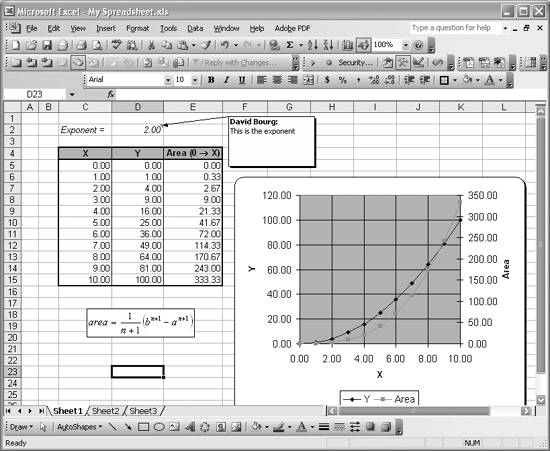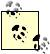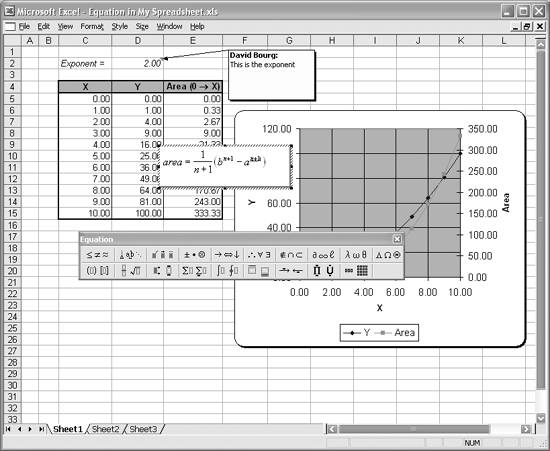Problem

Solution

Aside from simply inserting text in cells adjacent to cells containing data or formulas, you can use comments or equation objects to document your spreadsheets.

Discussion

Figure 1-23 shows a sample spreadsheet that includes a comment and an equation object documenting parts of the spreadsheet.

Figure 1-23. Spreadsheet with comment and equationThe comment is the rectangle containing the text "David Bourg: This is the exponent," with an arrow pointing to cell D2. The equation object is the rectangle containing the mathematical expression for the area calculation. Both comments and equations are useful devices for documenting your spreadsheets. I often use comments to leave myself notes or reminders or to-do lists within my spreadsheets. I use equations to document formulas used in my spreadsheets that will be used by others or included in reports or other presentations. Equations in standard mathematical form are much clearer and far easier to comprehend than cell formulas containing a bunch of cell references and operators all strung together.

To add a comment, first select the cell to which you want the comment attached, then select InsertComment from the main menu bar. A comment box will appear (with input focus, so you can immediately begin typing your comment). When youre done typing, use the mouse to select where on the spreadsheet you want the comment box to be placed. To edit an existing comment, select the cell containing the comment and select Insert [Image_Link]/images/U2192.jpg border=0> Edit Comment from the main menu bar. This will give input focus to the comment, allowing you to edit its message. To delete a comment, select the cell containing the comment and right-click to reveal a pop-up menu where you can select Delete Comment to actually delete the comment.You can also use the Reviewing toolbar (by selecting View [Image_Link]/images/U2192.jpg border=0> Toolbars [Image_Link]/images/U2192.jpg border=0> Reviewing) to access comment features such as adding, editing, deleting, or temporarily hiding comments.

To add an equation, you need to use the Microsoft Equation Editor to insert an equation object into your spreadsheet. Select Insert [Image_Link]/images/U2192.jpg border=0> Object... to show the Object Dialog box. From the list of Object Types in this dialog box, choose Microsoft Equation 3.0. When you press OK, the dialog will close, returning you to the spreadsheet in equation-editing mode (see Figure 1-24).

The equation rectangle will appear, allowing you to enter an equation using the equation toolbar shown in Figure 1-24. You can type text in the equation using the keyboard. To insert mathematical symbols, select them from the equation toolbar. Use the arrow keys on the keyboard to navigate an equation while editing. The Equation Editor is a bit cumbersome at first; I suggest you try it and practice until you get the hang of it. When you're done entering an equation, simply click anywhere in your spreadsheet to close the Equation Editor. You can click and drag the resulting equation to place it anywhere in your spreadsheet. To edit an existing equation, select it and then right-click on it to reveal a pop-up menu. Select Equation Object [Image_Link]/images/U2192.jpg border=0> Edit from this pop-up menu to edit the equation. To delete an equation, simply select it and press the Delete key on your keyboard.

Figure 1-24. Editing an equationThere's an alternative to editing equations directly on your spreadsheet as illustrated in Figure 1-24. If you select an equation object and then right-click to open the pop-up menu, you can select the Equation Object [Image_Link]/images/U2192.jpg border=0> Open menu option. This will open a new Equation Editor window showing your equation along with the Equation Editor toolbar. You can edit your equation here and select File [Image_Link]/images/U2192.jpg border=0> Exit to return to your spreadsheet.

The Equation Editor also contains a help menu option. Select Help [Image_Link]/images/U2192.jpg border=0> Equation Editor Help Topics to open the Equation Editor help file and read more about using the Equation Editor.The Equation Editor discussed here is the same one that's accessible from Microsoft Word . You can insert equations in your Word documents in the same way that you add equations to a spreadsheet, and you don't have to have Excel open in order to do so. This is useful for preparing technical reports in Word.Excel Scientific and Engineering Cookbook (Cookbooks (OReilly))
ISBN: 0596008791
EAN: 2147483647
Year: N/A
Pages: 206
Authors: David M Bourg